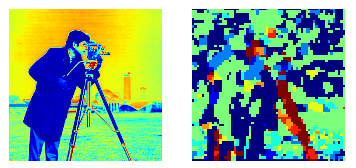# [PYTHON] About HOG output of Scikit-Image

#### `skimage.feature.hog (http://scikit-image.org/docs/dev/api/skimage.feature.html#hog)Extracts HOG features from the input image, but the output result is 1d-It is an array, and there is a problem that I do not know what it is. https://github.com/holtzhau/scikits.image/blob/master/skimage/feature/hog.You can read the code in py to see how to interpret this array.`

``````

### Important input arguments

--```orientations```: Histogram bins (default = 9)
--``` pixels_per_cell```: Cell size (default = (8,8))
--``` cells_per_block```: Number of cells per block (default = (3, 3))

### Take a look at the output

Line 180

#### **`hog.py`**
```py

return normalised_blocks.ravel()
``````

I wanted you to return it without raveling here. Looking at the declaration of normalised_blocks,

Line 160

#### `hog.py`

``````
n_blocksx = (n_cellsx - bx) + 1
n_blocksy = (n_cellsy - by) + 1
normalised_blocks = np.zeros((n_blocksx, n_blocksy, bx, by, orientations))
``````

The variables used here are declared around line 100

#### `hog.py`

``````
sx, sy = image.shape
cx, cy = pixels_per_cell
bx, by = cells_per_block

n_cellsx = int(np.floor(sx // cx))  # number of cells in x
n_cellsy = int(np.floor(sy // cy))  # number of cells in y
``````

So, the 1-d array (let's say `` `retval```) obtained by turning hog is

``````retval.reshape((n_blocksx, n_blocksy, bx, by, orientations))
``````

If you give it as, you can return it to its original shape.

### Usage example

For example, the maximum gradient direction for each block can be visualized.

#### `visualize_argmaxhog.py`

``````
from skimage.feature import hog
from skimage.data import camera
import matplotlib.pyplot as plt

img = camera()
orientations = 9
pixels_per_cell = (8, 8)
cells_per_block = (3, 3)

h = hog(img, orientations=orientations, pixels_per_cell=pixels_per_cell, cells_per_block=cells_per_block)

sx, sy = img.shape[:2]
cx, cy = (8, 8)
bx, by = (3, 3)
n_cellsx = int(np.floor(sx // cx))  # number of cells in x
n_cellsy = int(np.floor(sy // cy))  # number of cells in y
n_blocksx = (n_cellsx - bx) + 1
n_blocksy = (n_cellsy - by) + 1

himg = h.reshape((n_blocksx * n_blocksy, bx, by, orientations))
vis = np.array([np.argmax(x.sum(axis=(0, 1))) for x in himg]).reshape((n_blocksx, n_blocksy))

plt.subplot(1, 2, 1)
plt.imshow(img)
plt.axis('off')
plt.subplot(1, 2, 2)
plt.imshow(vis, interpolation='nearest')
plt.axis('off')

``````